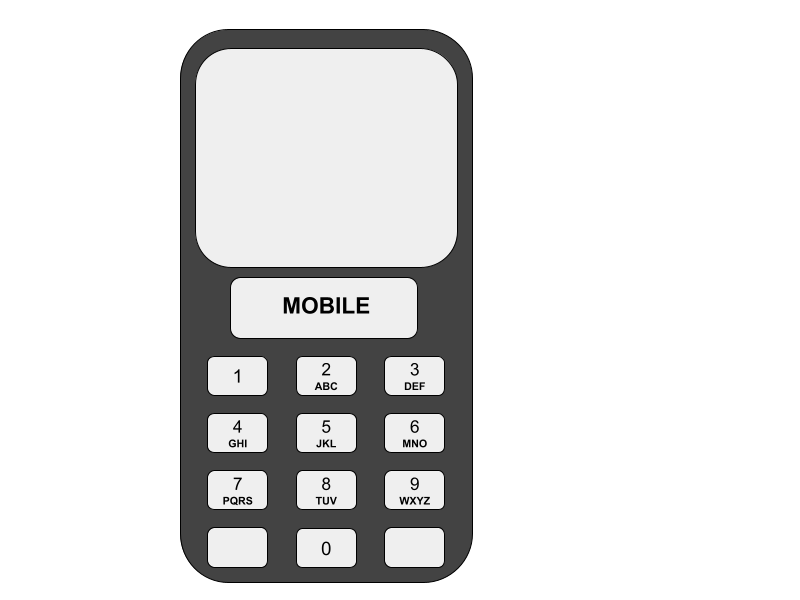New update is available. Click here to update.
Last Updated: 10 Mar, 2021
##### Phone Code
Hard
Problem statement#### One day ninja saw his father using an old phone with a number pad and thought of a scenario. She saw that the phone had a numeric keypad and each number was mapped to some alphabets as shown in the figure below. She has a list of valid words. She wants to create an algorithm that would take a given sequence of numbers as input and return a list of matching words that are also present in her list of valid words.##### Note:
`````` You can convert the list of valid words into any data structure you desire.
``````
##### For example :
``````If the sequence of number = 2633, and the list of valid words = [ride, used, code, tree, boed],
Then you would print “code” and “boed” as they can be formed by using the digit sequence and they are also present in the list of valid words.
``````
##### Input Format:
``````The first line contains an integer ‘T’ which denotes the number of test cases or queries to be run. Then the test cases are as follows.
The first and line of each test case consist of the sequence of numbers.
The second line of each test case consists of ‘W’, which denotes the number of given valid words.
The third and final line of each test case consists of the list of ‘W’ valid words.
``````
##### Output Format:
``````For each test case, print the list of words that can be formed using the given sequence of numbers and are also present in the given list of valid words.

Print the output of each test case in a separate line.
``````
##### Note:
``````You don’t need to print anything; It has already been taken care of. You just need to complete the given function.
``````
##### Constraints:
``````1 <= T <= 10
1 <= Sequence <= 10
1 <= W <= 200

where ‘T’ is the number of test cases, “sequence” is the length of the given sequence of numbers, and “W”, is the number of valid words each test case can have.

Time limit: 1 sec
``````Approaches

## 01ApproachThis is the most basic approach and we will simply try every possible value for each digit in the sequence with all other possible values.

• We start by taking the first digit of the sequence and run through all the characters that map to that digit.
• For every character, we then add it to a variable let’s say “pre” and recurse, by passing the “pre” downwards.
• We do this until we run out of characters and then simply print the variable “pre” that would by now contain the full word, if we can find it in the vector of valid words, meaning if it is a valid word.

Algorithm:

• We start by mapping the digits with their corresponding letters.
• For the main function, we start with creating a vector that will store our final “result”
• Create a recursive function with the base case:
• If there are no more digits to check, meaning if the length of the word is equal to the length of the sequence:
• Add the word “pre”, which is the current word to the “result” vector
• For the main function, we get characters that match the given digit with the help of the precomputed mapping of digits with their corresponding letters.
• Recursively call for the rest of the digits until we reach the end of the sequence.
• Check for “pre” in the list of valid words:
• If found:
• Push it into “result”.
• Finally, return the result vector.